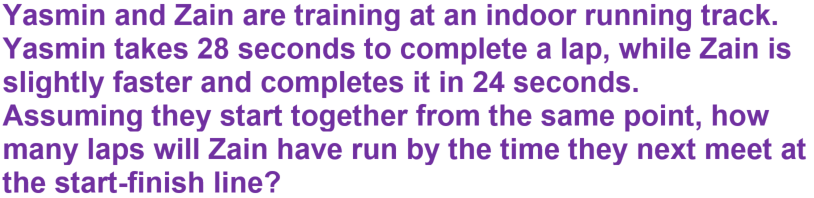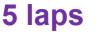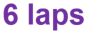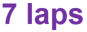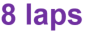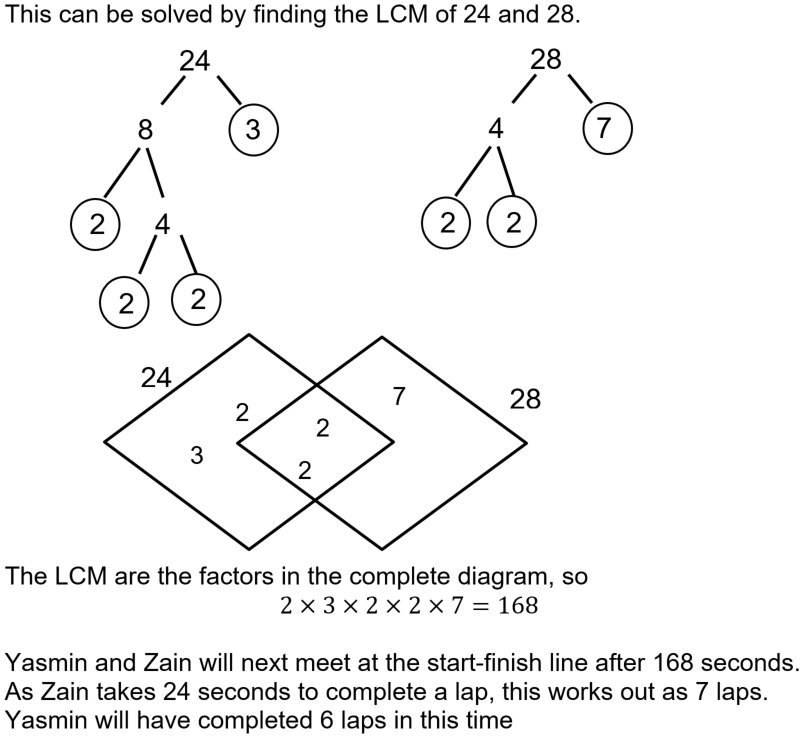Test your maths skills in this quiz.

# HCF and Lowest Common Multiple (F)

Do you know your factors from your multiples? Can you list the first 10 prime numbers? Being able to find the Highest Common Factor (HCF) and Lowest Common Multiple (LCM) of two numbers will help you when it comes to working with fractions, but there are also many real-life applications that use them. See how good you are in this GCSE Maths quiz.

When dealing with factors and multiples, the first thing to get right is which way round they go! A FACTOR goes into a number, and a MULTIPLE comes out of a number. All numbers have at least 2 factors – 1 and the number itself. You can list the factors of a number by writing them in pairs. The multiples of a number are simply the times tables of that number.

You can also break a number down into its PRIME factors. For example, 12 can be written as 22 x 3. This is a useful technique for large numbers, particularly if finding HCF or LCM of 2 numbers.

Questions involving HCF and LCM will involve 2 or more numbers. The HCF asks you to find the biggest (highest) factor that belongs (is common) to both numbers. The LCM wants the smallest (lowest) number that is a multiple of both numbers.

It is easy to mix up HCF and LCM. The HCF will be smaller than or equal to the smaller number, and the LCM will be bigger than or equal to the largest number. Try it for yourself!

Question 1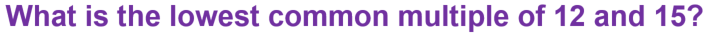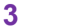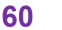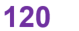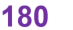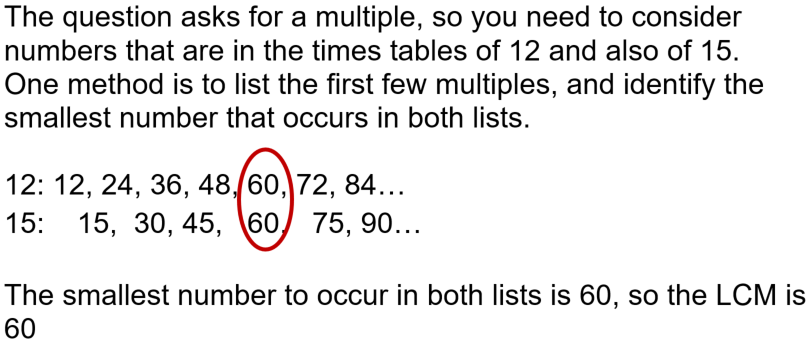Question 2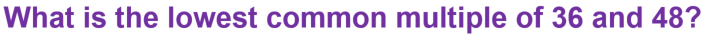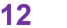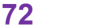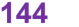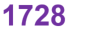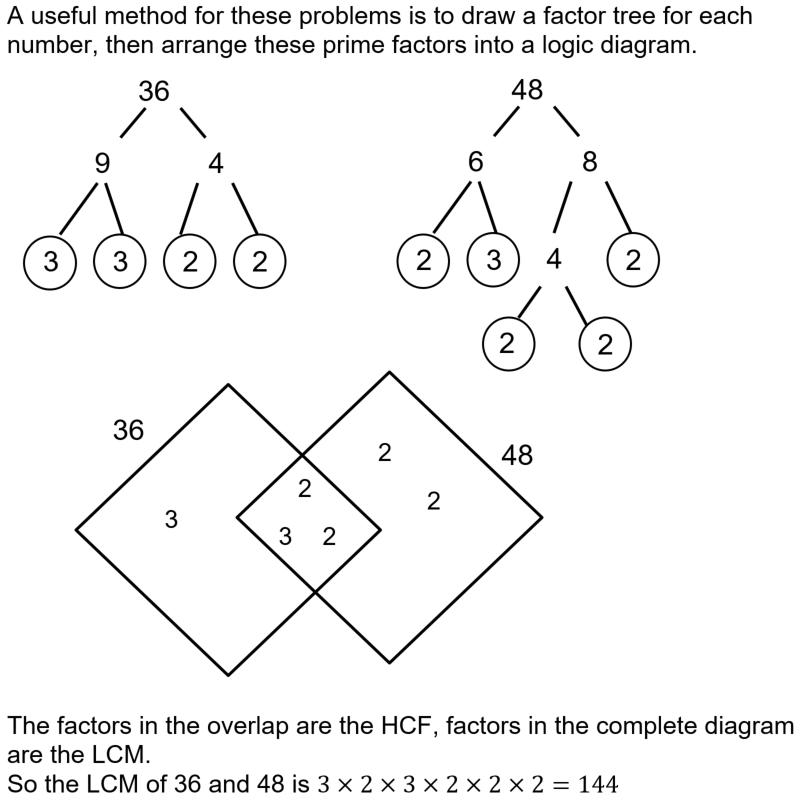Question 3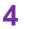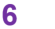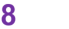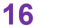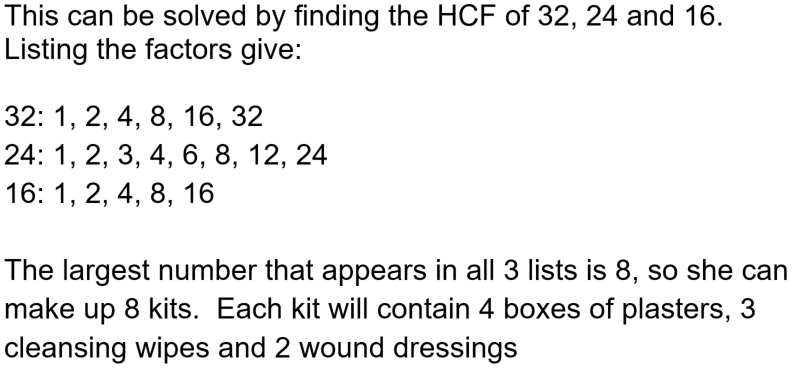Question 4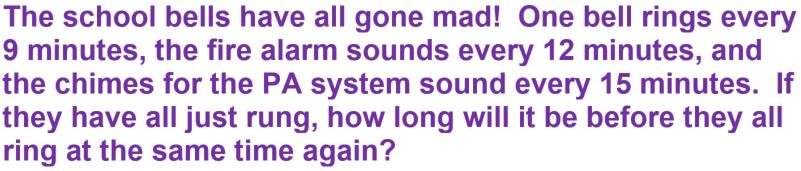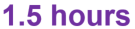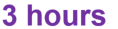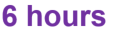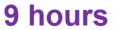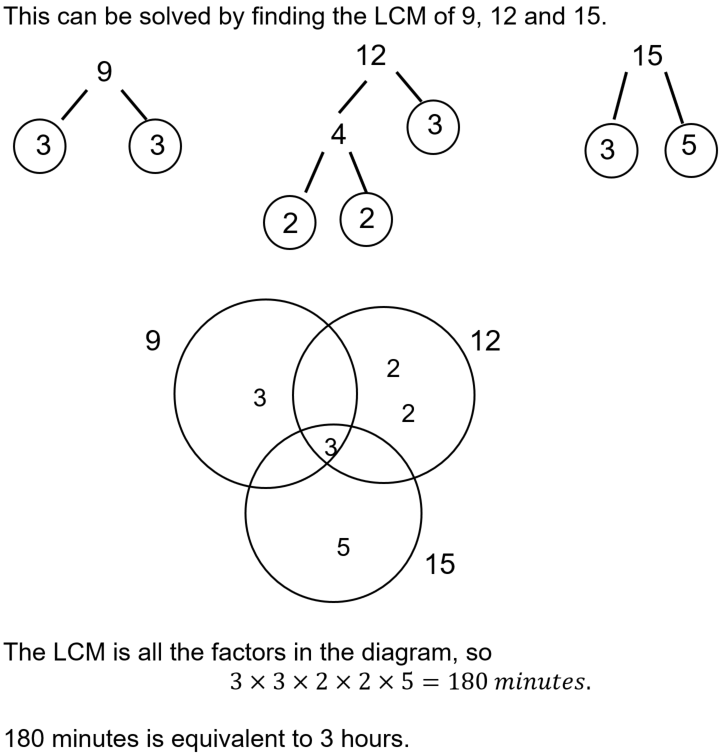Question 5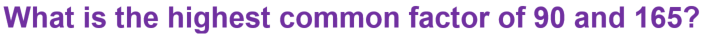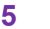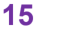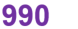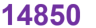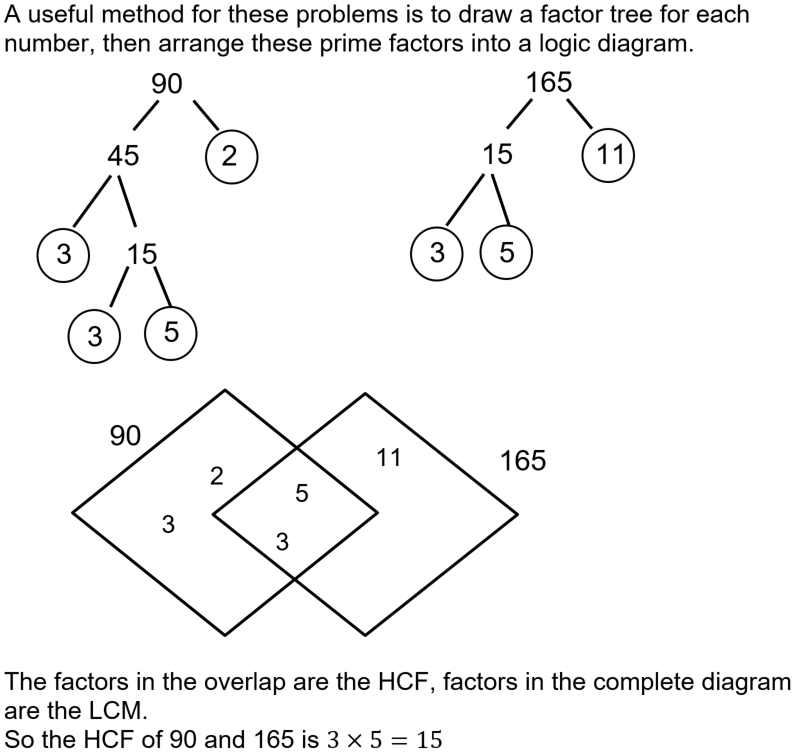Question 6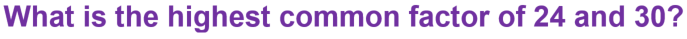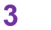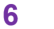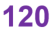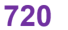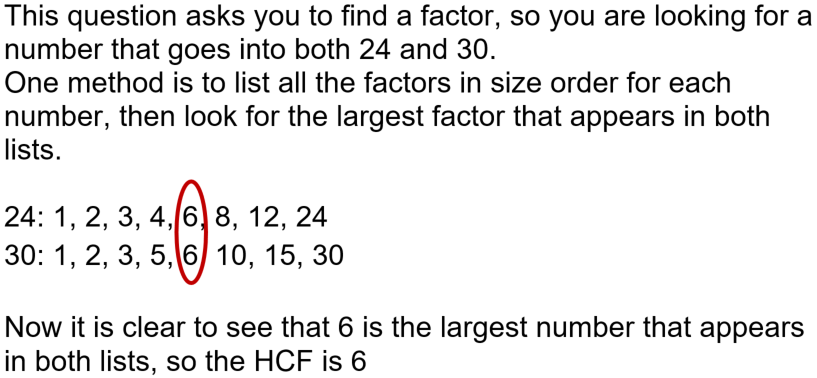Question 7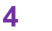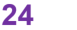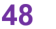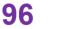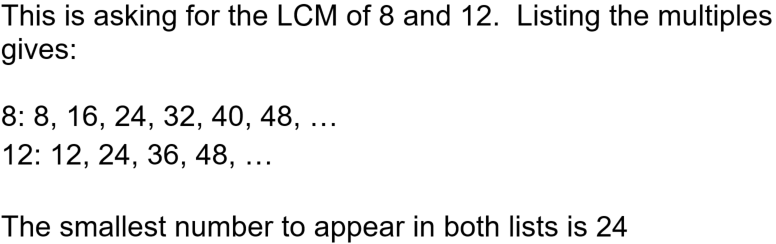Question 8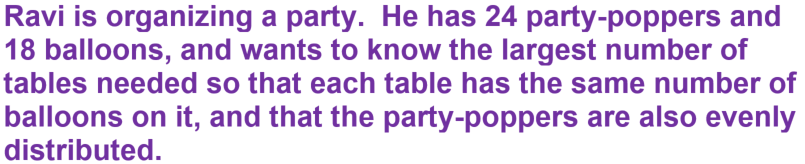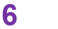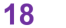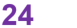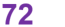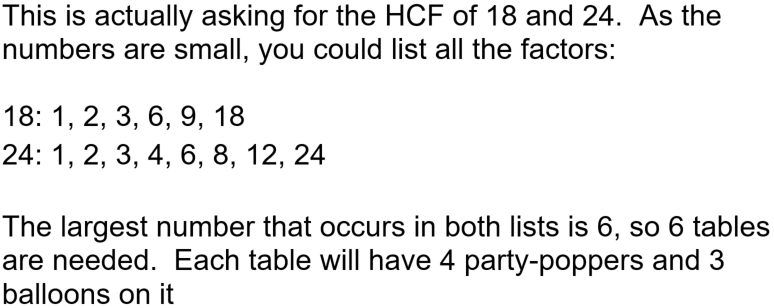Question 9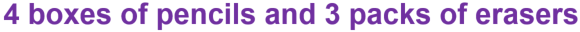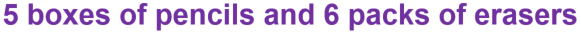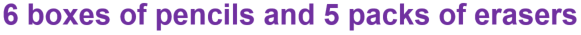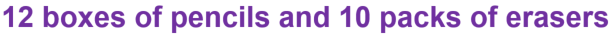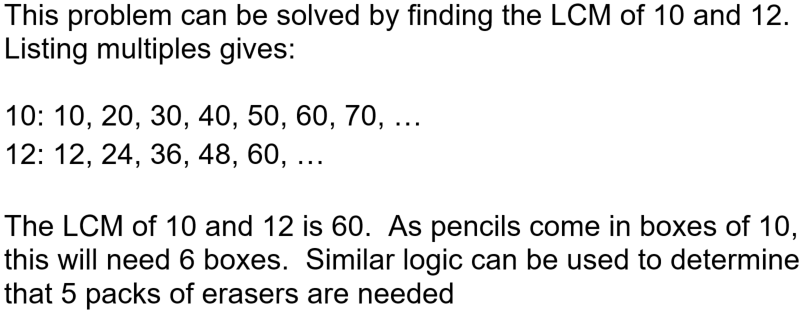Question 10